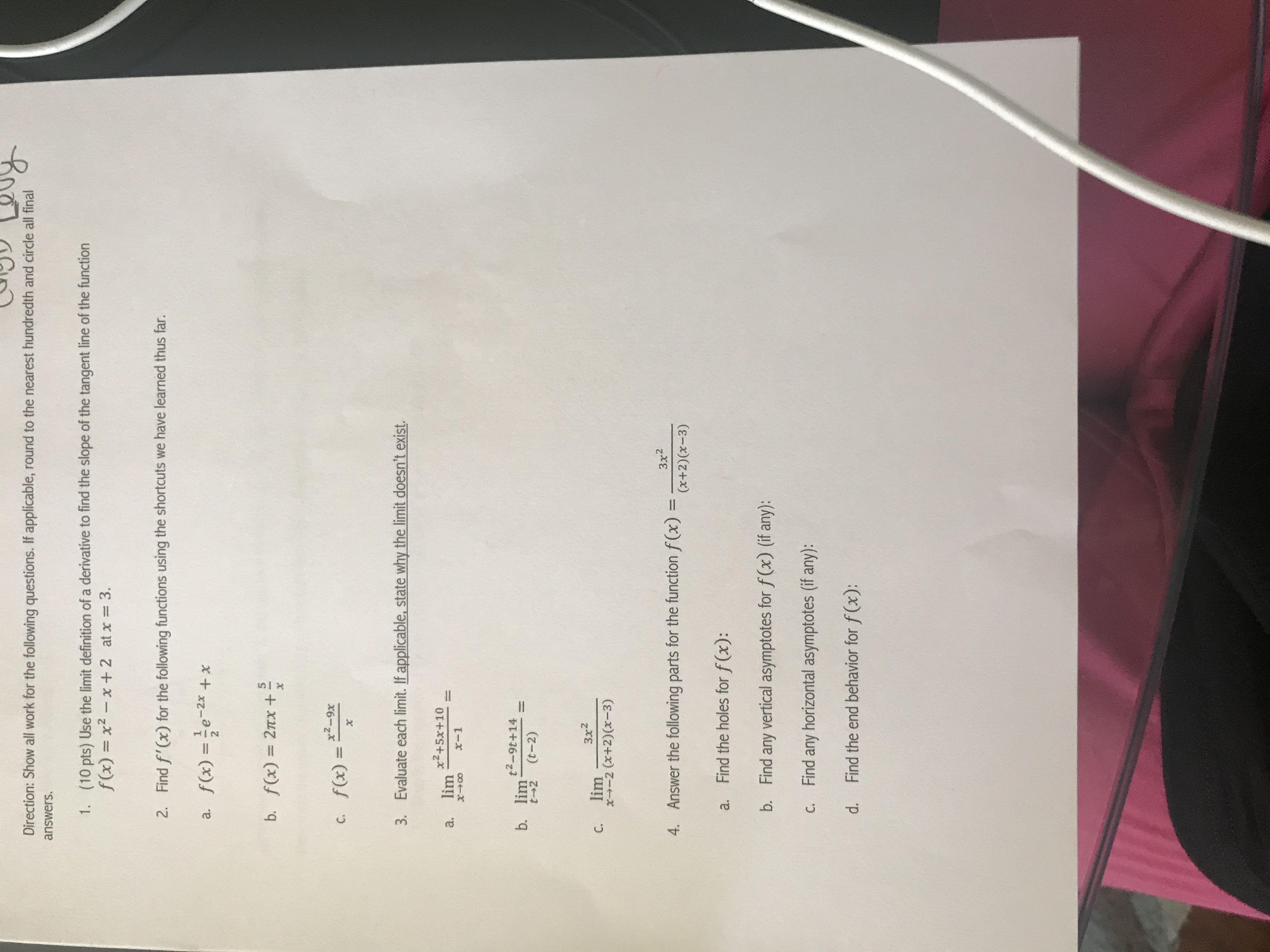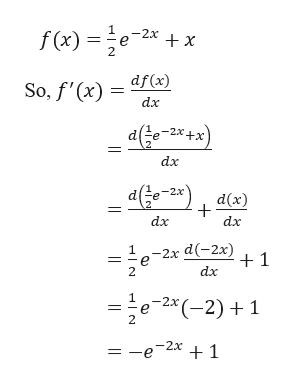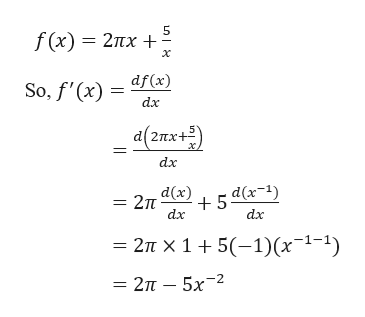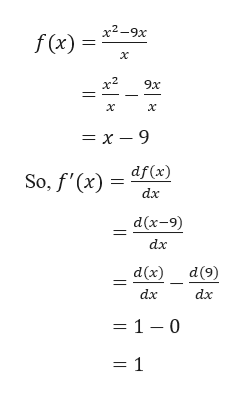# Direction: Show all work for the following questions. If applicable, round to the nearest hundredth and circle all finalanswers.(10 pts) Use the limit definition of a derivative to find the slope of the tangent line of the functionf(x) x2-1.x 2 at x3.Find f'(x) for the following functions using the shortcuts we have learned thus far.2.f(x)=e-x.а.b. f(x) 2x +f(x)=2-9xC.XEvaluate each limit. If applicable, state why the limit doesn't exist.3.x2+5x+10limа.1x-1t2-9t+14b. limt--2(t-2)3x2limС.x-2 (x+2)(x-3)Зx24. Answer the following parts for the function f (x) (x+2)(x-3)Find the holes for f(x):a.Find any vertical asymptotes for f (x) (if any):b.Find any horizontal asymptotes (if any):С.Find the end behavior for f(x):d.n

Question
94 viewshelp_outlineImage TranscriptioncloseDirection: Show all work for the following questions. If applicable, round to the nearest hundredth and circle all final answers. (10 pts) Use the limit definition of a derivative to find the slope of the tangent line of the function f(x) x2- 1. x 2 at x 3. Find f'(x) for the following functions using the shortcuts we have learned thus far. 2. f(x)=e-x. а. b. f(x) 2x + f(x)=2-9x C. X Evaluate each limit. If applicable, state why the limit doesn't exist. 3. x2+5x+10 lim а. 1 x-1 t2-9t+14 b. lim t--2 (t-2) 3x2 lim С. x-2 (x+2)(x-3) Зx2 4. Answer the following parts for the function f (x) (x+2)(x-3) Find the holes for f(x): a. Find any vertical asymptotes for f (x) (if any): b. Find any horizontal asymptotes (if any): С. Find the end behavior for f(x): d. n fullscreen
check_circle

star
star
star
star
star
1 Rating
Step 1

Question No. 2

Part a

Given,help_outlineImage Transcriptionclose1 -2x + X f(x)2xx So, f'(x)df (x) dx dx + dx dx d(-2x) 1 dx 2 2x *(-2)1 2 | fullscreen
Step 2

Part b

Given,help_outlineImage Transcriptionclose5 f (x) — 2пх + х So, f'(x) df (x) dx a(2пх+5) dx — 2п ах) , 5 dx-1) dx dx 2п х 1 + 5(-1)(x 1-1) - 2п — 5х-2 fullscreen
Step 3

Part c

Given...help_outlineImage Transcriptionclosef(x) = x2-9x х 9x х = x - 9 So, f'(x) df(x) dx d(x-9) dx d(x) d(9) dx dx = 1 - 0 = 1 | fullscreen

### Want to see the full answer?

See Solution

#### Want to see this answer and more?

Solutions are written by subject experts who are available 24/7. Questions are typically answered within 1 hour.*

See Solution
*Response times may vary by subject and question.
Tagged in

### Derivative